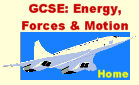Forces : pushes and pulls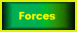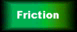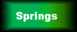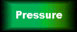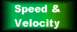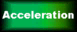We can't actually see forces, but we see their effect on objects. We see the Moon orbit the Earth, objects fall to the ground, and birds fly - all of these are because of forces.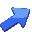A force is a push or a pull. We measure forces in "Newtons" (N) named after Sir Isaac Newton (1642 - 1727). 1 Newton isn't a very big force: it's about the weight of an apple. Forces are vectors, because the direction is important.

 Forces can change:- the speed of an object the direction that an object is moving in the shape of an object.
 Gravity is a force that acts towards the centre of the Earth. This means that, wherever you are in the world, "down" is always towards the ground - even though your "down" isn't the same direction as anybody else's. The gravitational pull of the Earth is what gives objects weight. Thus weight is a force - it's how hard the Earth is pulling on an object. The Earth pulls on every kilogramme with a force of ten Newtons. We say that the Earth's gravitational field strength (at ground level) is 10 Newtons per kilogramme (10 N/kg) (Actually, it's more like 9.81, but for GCSE we usually call it 10.) In other words, an object with a mass of 1kg has a weight of 10N.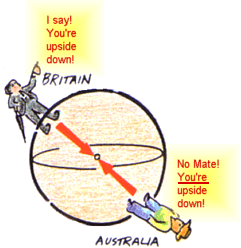Gravity is a very weak force, you need a very large mass in order to get a noticeable gravitational pull. An odd thing about gravity - it always attracts objects and never repels them. Gravity Questions (click on the question to reveal the answers)

Sir Isaac Newton came up with three "Laws of Motion" back in the 17th century, which we still find useful today. For the moment, we need:-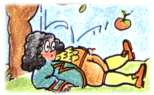Newton's First Law,
says that if the forces on an object are in balance, the object's speed and direction of motion won't change.
(In other words, if you leave it alone, it'll carry on doing whatever it was doing already.)

### If the forces on an object are in balance, then the object's velocity is constant.

This means that if it's not moving, it'll stay still; or if it is moving, it'll continue in a straight line at a constant speed .

What if the forces aren't in balance? See Acceleration page

 Here is a picture of a parchustist, descending at a constant speed. What forces are acting? What can you say about the size of the forces? Click on the picture to see the answer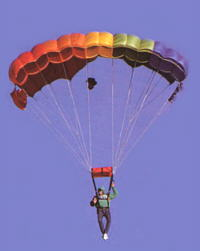When several forces act on an object, we can add them together to find out what's going to happen. The combined effect of all the forces on an object is called the resultant force. For GCSE, you'll usually only be asked to deal with forces which are all in a straight line. This makes it really easy! Question: if I push a car with a force of 500N, and somebody else pushes the other way with a force of 400N, what is the resultant force on the car? Click here for answer. If these pictures aren't moving, click on "Refresh"/"Reload"Advanced:If the forces are not in a straight line, we must use other techniques, such as a "triangle of forces", to find the resultant. If this picture isn't moving, click on "Refresh"/"Reload"Newton's Third Law, says that every action has an equal and opposite reaction. In other words, when you push or pull something, it pushes or pulls back at you. Forces are two-way affairs, they're about the way that objects affect each other. For example, you're pushing down on your chair with a force of, maybe, 500N, and the chair is pushing back with a force of 500N. The forces are balanced, and as you're already sat still, you stay that way.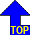of page